# Selina Solutions Concise Mathematics Class 6 Chapter 14: Fractions Exercise 14(C)

Selina Solutions Concise Mathematics Class 6 Chapter 14: Fractions Exercise 14(C) has 100% answers based on addition and subtraction of fractions, with illustrations. Solutions are designed by expert teachers in an interactive manner, helping students to clear their doubts about the concept quickly. Following these solutions, students surely enhance their problem solving abilities within a short period. To score more marks in exams, download Selina Solutions Concise Mathematics Class 6 Chapter 14 Fractions Exercise 14(C), PDF links available below.

## Selina Solutions Concise Mathematics Class 6 Chapter 14: Fractions Exercise 14(C) Download PDF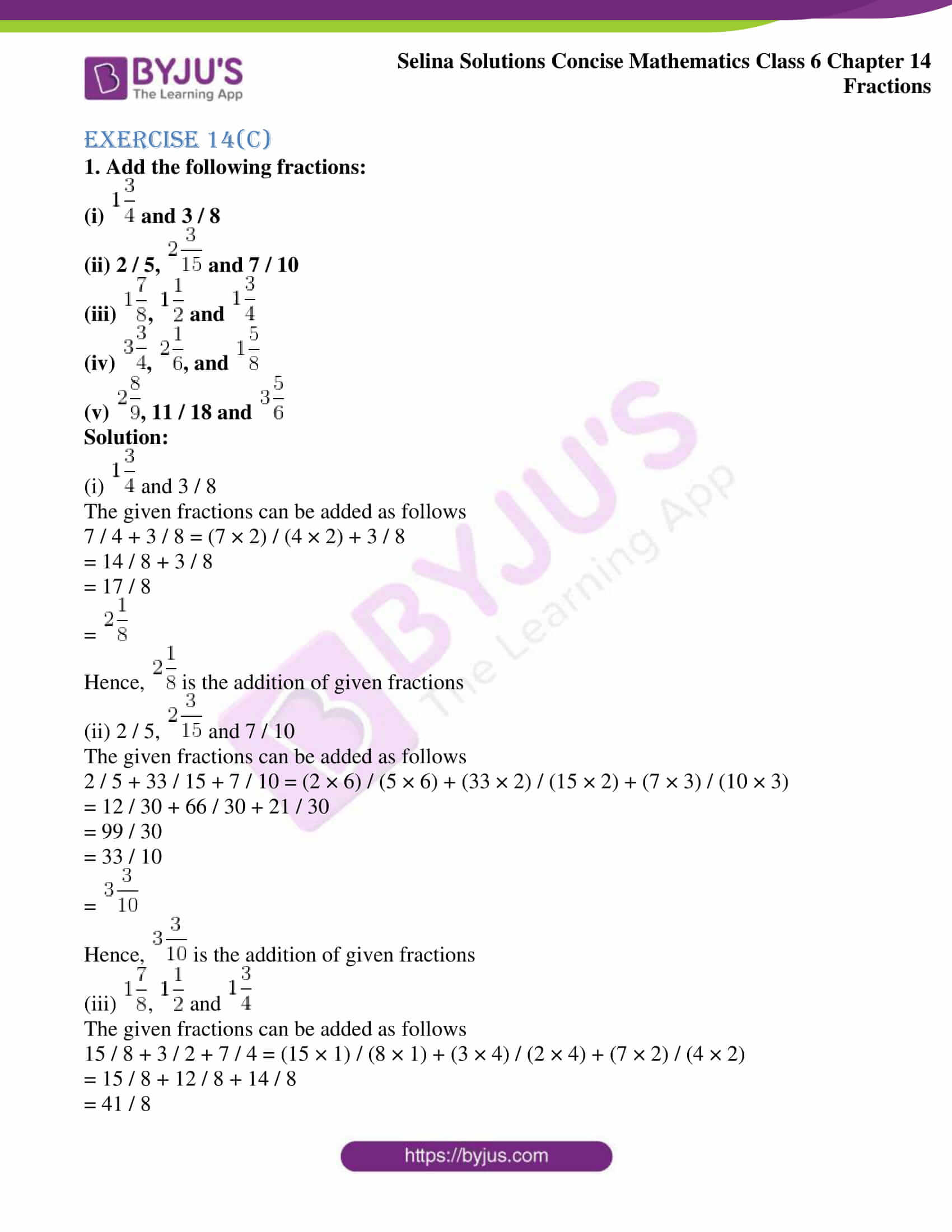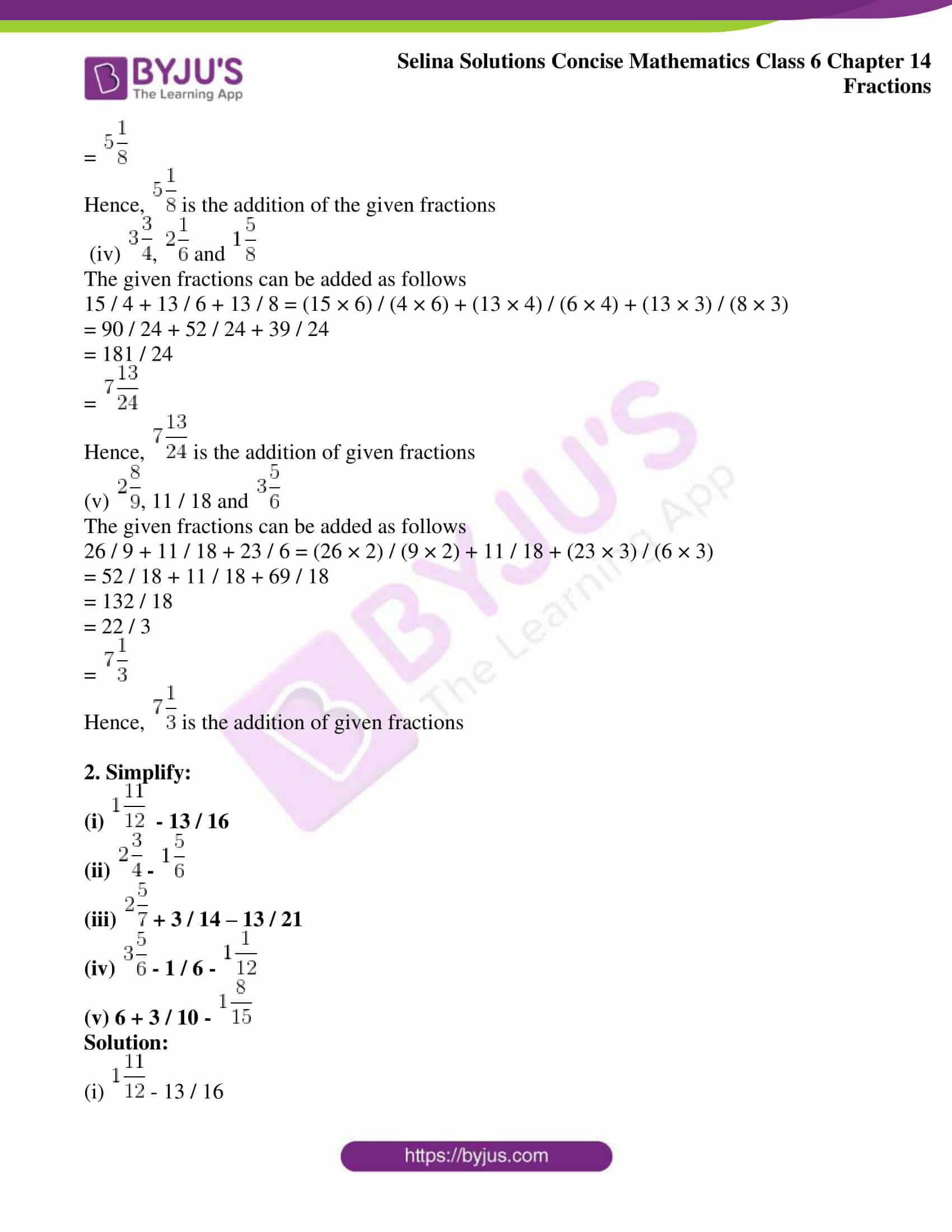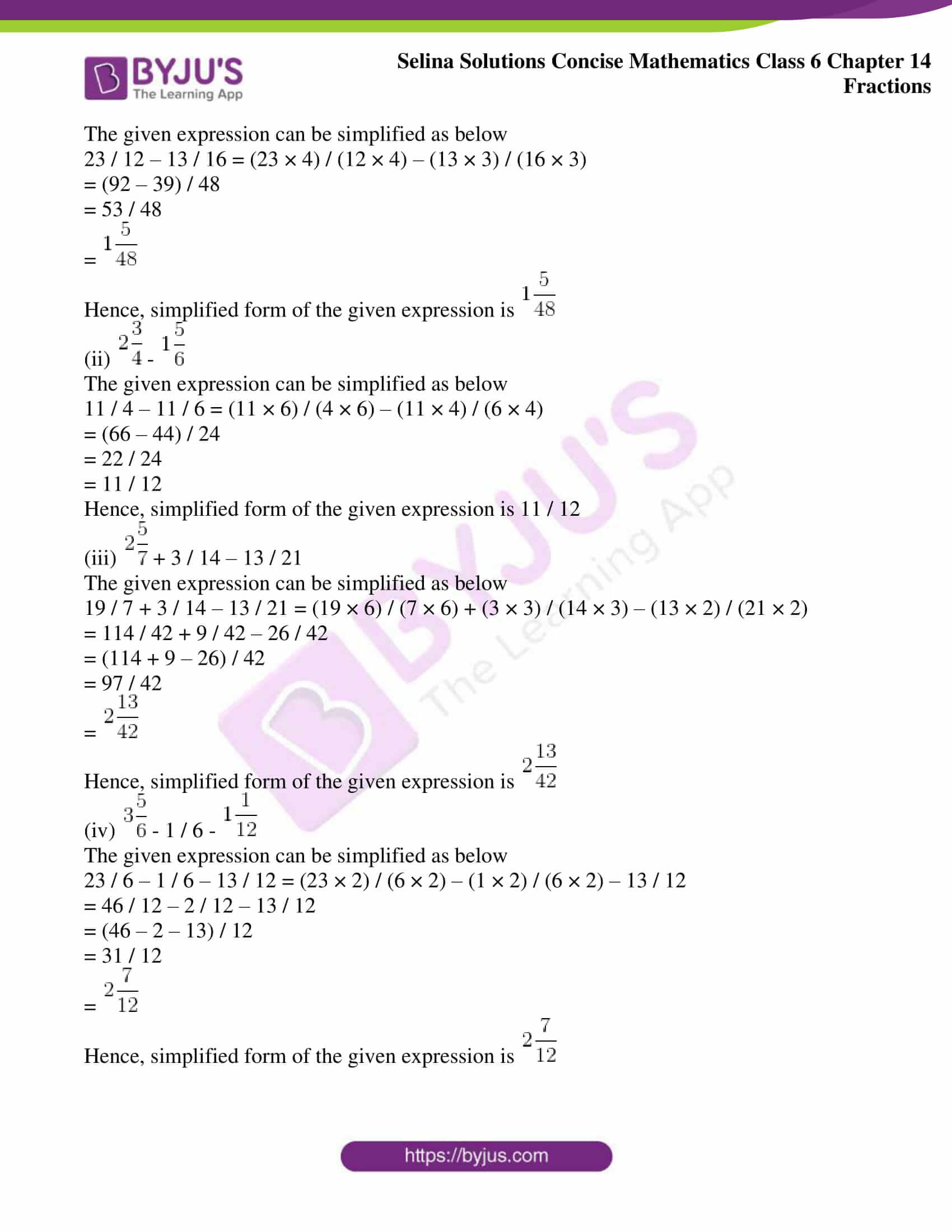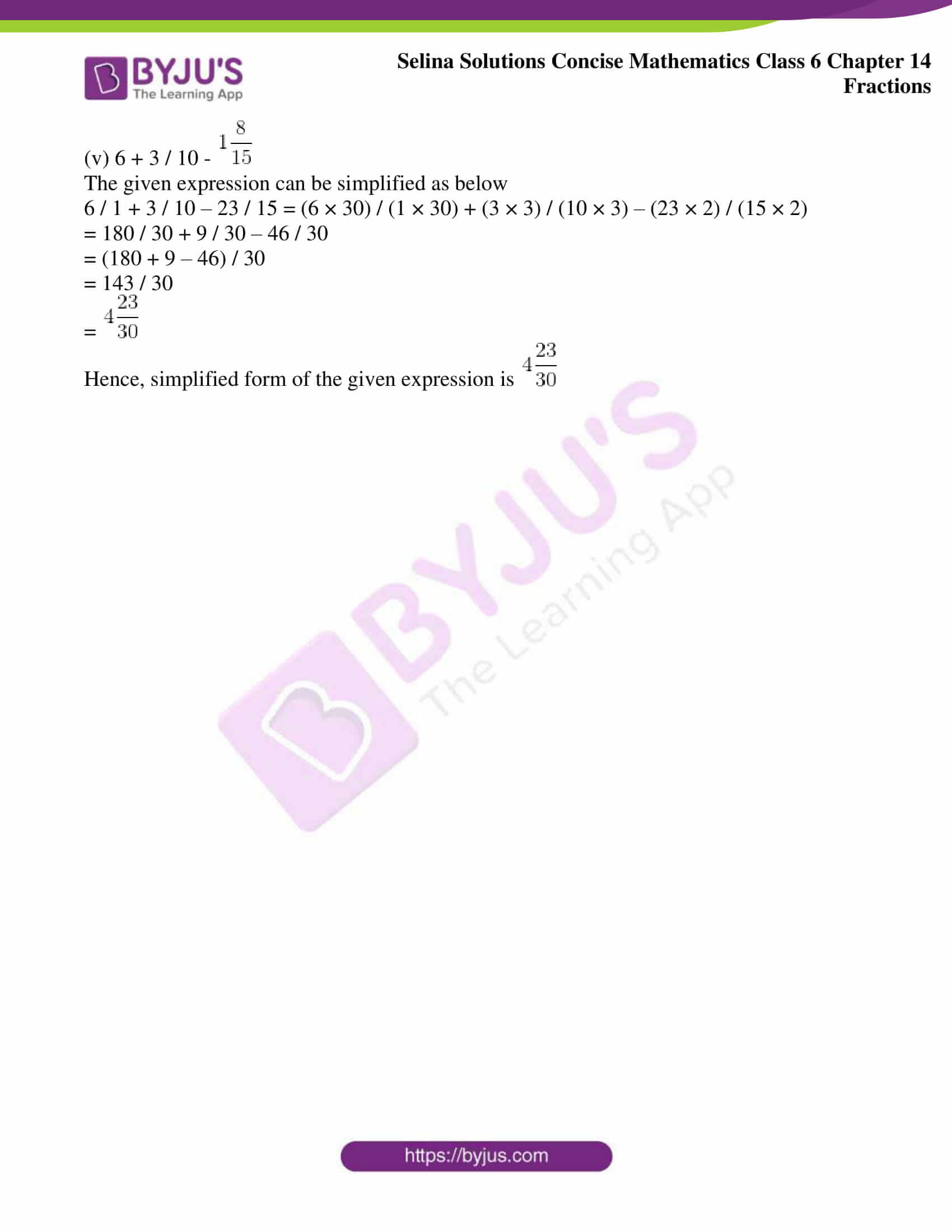### Access other exercises of Selina Solutions Concise Mathematics Class 6 Chapter 14: Fractions

Exercise 14(A) Solutions

Exercise 14(B) Solutions

Exercise 14(D) Solutions

Exercise 14(E) Solutions

### Access Selina Solutions Concise Mathematics Class 6 Chapter 14: Fractions Exercise 14(C)

Exercise 14(C)

(i)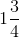and 3 / 8

(ii) 2 / 5,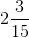and 7 / 10

(iii)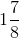,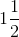and(iv)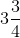,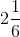, and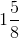(v)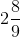, 11 / 18 and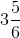Solution:

(i)and 3 / 8

The given fractions can be added as follows

7 / 4 + 3 / 8 = (7 × 2) / (4 × 2) + 3 / 8

= 14 / 8 + 3 / 8

= 17 / 8

=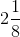Hence,is the addition of given fractions

(ii) 2 / 5,and 7 / 10

The given fractions can be added as follows

2 / 5 + 33 / 15 + 7 / 10 = (2 × 6) / (5 × 6) + (33 × 2) / (15 × 2) + (7 × 3) / (10 × 3)

= 12 / 30 + 66 / 30 + 21 / 30

= 99 / 30

= 33 / 10

=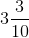Hence,is the addition of given fractions

(iii),andThe given fractions can be added as follows

15 / 8 + 3 / 2 + 7 / 4 = (15 × 1) / (8 × 1) + (3 × 4) / (2 × 4) + (7 × 2) / (4 × 2)

= 15 / 8 + 12 / 8 + 14 / 8

= 41 / 8

=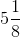Hence,is the addition of the given fractions

(iv),andThe given fractions can be added as follows

15 / 4 + 13 / 6 + 13 / 8 = (15 × 6) / (4 × 6) + (13 × 4) / (6 × 4) + (13 × 3) / (8 × 3)

= 90 / 24 + 52 / 24 + 39 / 24

= 181 / 24

=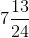Hence,is the addition of given fractions

(v), 11 / 18 andThe given fractions can be added as follows

26 / 9 + 11 / 18 + 23 / 6 = (26 × 2) / (9 × 2) + 11 / 18 + (23 × 3) / (6 × 3)

= 52 / 18 + 11 / 18 + 69 / 18

= 132 / 18

= 22 / 3

=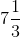Hence,is the addition of given fractions

2. Simplify:

(i)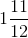– 13 / 16

(ii)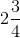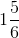(iii)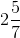+ 3 / 14 – 13 / 21

(iv)– 1 / 6 –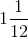(v) 6 + 3 / 10 –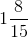Solution:

(i)– 13 / 16

The given expression can be simplified as below

23 / 12 – 13 / 16 = (23 × 4) / (12 × 4) – (13 × 3) / (16 × 3)

= (92 – 39) / 48

= 53 / 48

=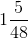Hence, simplified form of the given expression is(ii)The given expression can be simplified as below

11 / 4 – 11 / 6 = (11 × 6) / (4 × 6) – (11 × 4) / (6 × 4)

= (66 – 44) / 24

= 22 / 24

= 11 / 12

Hence, simplified form of the given expression is 11 / 12

(iii)+ 3 / 14 – 13 / 21

The given expression can be simplified as below

19 / 7 + 3 / 14 – 13 / 21 = (19 × 6) / (7 × 6) + (3 × 3) / (14 × 3) – (13 × 2) / (21 × 2)

= 114 / 42 + 9 / 42 – 26 / 42

= (114 + 9 – 26) / 42

= 97 / 42

=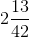Hence, simplified form of the given expression is(iv)– 1 / 6 –The given expression can be simplified as below

23 / 6 – 1 / 6 – 13 / 12 = (23 × 2) / (6 × 2) – (1 × 2) / (6 × 2) – 13 / 12

= 46 / 12 – 2 / 12 – 13 / 12

= (46 – 2 – 13) / 12

= 31 / 12

=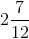Hence, simplified form of the given expression is(v) 6 + 3 / 10 –The given expression can be simplified as below

6 / 1 + 3 / 10 – 23 / 15 = (6 × 30) / (1 × 30) + (3 × 3) / (10 × 3) – (23 × 2) / (15 × 2)

= 180 / 30 + 9 / 30 – 46 / 30

= (180 + 9 – 46) / 30

= 143 / 30

=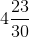Hence, simplified form of the given expression is